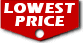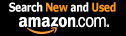# Fourier Series (Dover Books on Mathematics) / Georgi P. Tolstov

List Price: \$18.95
Our Price: \$11.95For Bulk orders
Quantity

Used Book Price:
/ Georgi P. Tolstov
Publisher: Dover Publications
Availability:In Stock.
Sales Rank: 264984
 ISBN-10: 0486633179ISBN-13: 9780486633176

Similar Books
 An Introduction to Information Theory: Symbols, Signals and Noise (Dover Books on Mathematics) Ordinary Differential Equations (Dover Books on Mathematics) Introduction to Topology: Third Edition (Dover Books on Mathematics) Introduction to Graph Theory (Dover Books on Mathematics) Linear Algebra (Dover Books on Mathematics) Partial Differential Equations for Scientists and Engineers (Dover Books on Mathematics) Probability Theory: A Concise Course (Dover Books on Mathematics) Number Theory (Dover Books on Mathematics) Mechanics (Dover Books on Physics) Fourier Transforms (Dover Books on Mathematics)

Richard A. Silverman's series of translations of outstanding Russian textbooks and monographs is well-known to people in the fields of mathematics, physics, and engineering. The present book is another excellent text from this series, a valuable addition to the English-language literature on Fourier series.
This edition is organized into nine well-defined chapters: Trigonometric Fourier Series, Orthogonal Systems, Convergence of Trigonometric Fourier Series, Trigonometric Series with Decreasing Coefficients, Operations on Fourier Series, Summation of Trigonometric Fourier Series, Double Fourier Series and the Fourier Integral, Bessel Functions and Fourier-Bessel Series, and the Eigenfunction Method and its Applications to Mathematical Physics. Every chapter moves clearly from topic to topic and theorem to theorem, with many theorem proofs given. A total of 107 problems will be found at the ends of the chapters, including many specially added to this English-language edition, and answers are given at the end of the text.
Richard Silverman's excellent translation makes this book readily accessible to mathematicians and math students, as well as workers and students in the fields of physics and engineering. He has also added a bibliography, containing suggestions for collateral and supplementary reading. 1962 edition.

Now you can buy Books online in USA,UK, India and more than 100 countries.
*Terms and Conditions apply
Disclaimer: All product data on this page belongs to.
No guarantees are made as to accuracy of prices and information.